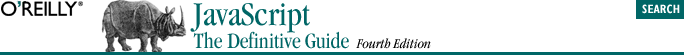home | O'Reilly's CD bookshelfs | FreeBSD | Linux | Cisco | Cisco Exam## 3.3. Boolean Values

`a == 4 `

This code tests to see if the value of the variable a is equal to the number 4. If it is, the result of this comparison is the boolean value true. If a is not equal to 4, the result of the comparison is false.

```if (a == 4)
b = b + 1;
else
a = a + 1; ```

This code checks if a equals 4. If so, it adds 1 to b; otherwise, it adds 1 to a.